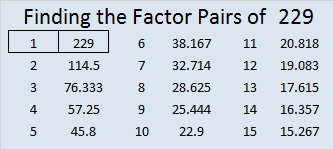# 229 and Level 4

• 229 is a prime number.
• Prime factorization: 229 is prime.
• The exponent of prime number 229 is 1. Adding 1 to that exponent we get (1 + 1) = 2. Therefore 229 has exactly 2 factors.
• Factors of 229: 1, 229
• Factor pairs: 229 = 1 x 229
• 229 has no square factors so its square root cannot be simplified. √229 ≈ 15.133How do we know that 229 is a prime number? If 229 were not a prime number, then it would be divisible by at least one prime number less than or equal to √229 ≈ 15.133. Since 229 cannot be divided evenly by 2, 3, 5, 7, 11, or 13, we know that 229 is a prime number.Excel file of puzzles and previous week’s factor solutions: 12 Factors 2014-09-08This site uses Akismet to reduce spam. Learn how your comment data is processed.